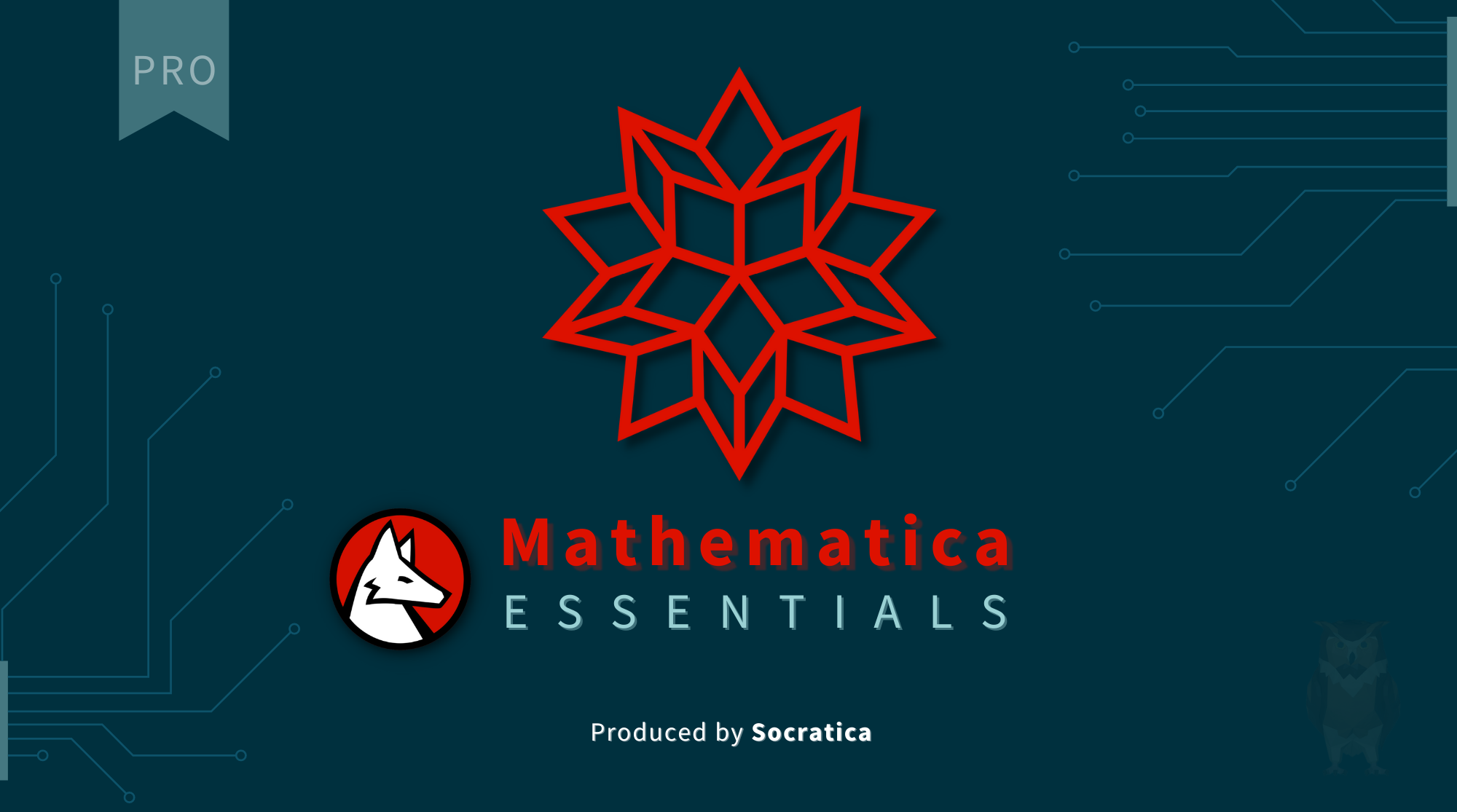Whats'
W

## Socratica's first pro course

MATHEMATICA ESSENTIALS

Mathematica Essentials is a comprehensive first course in Mathematica. The name 'Mathematica' may sound like the program is just for mathematics; but it can be used in ANY computational field: financial analysis, machine learning, data visualization, bioinformatics, speech recognition, and much, much more.We will also cover many essential mathematical applications: solving equations, numerical methods, calculus, modular arithmetic, and much more. While doing so, we will visit the essential features of the Wolfram Language - the language that lies at the heart of Mathematica, Wolfram | One, and other products from Wolfram Research.You can download sample notebooks, practice data, puzzles and more from our Wolfram github repository at: github.com/socratica/wolfram

INCLUDED IN THE COURSE:

20 LESSONS
QUICK TIPS
EXAMPLES
GITHUB REPOSITORY